# 法线贴图

Note## 法线贴图

vec3 rgb_normal = normal * 0.5 + 0.5; // 从 [-1,1] 转换至 [0,1]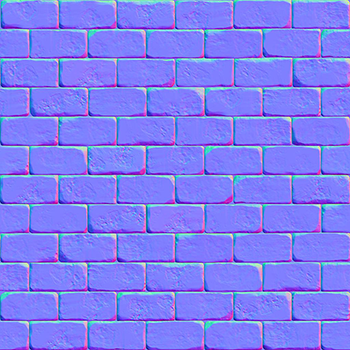uniform sampler2D normalMap;

void main()
{
// 从法线贴图范围[0,1]获取法线
normal = texture(normalMap, fs_in.TexCoords).rgb;
// 将法线向量转换为范围[-1,1]
normal = normalize(normal * 2.0 - 1.0);

[...]
// 像往常那样处理光照
}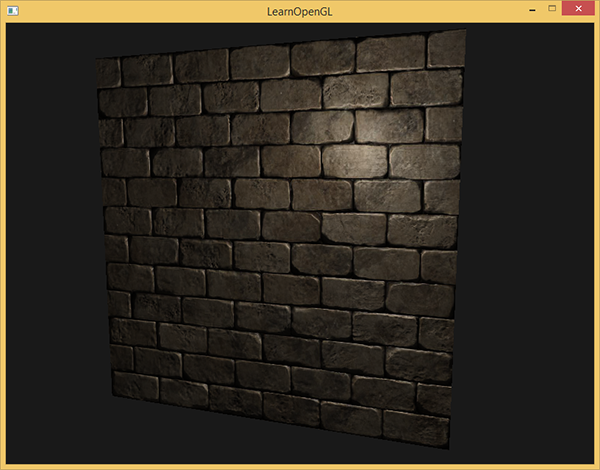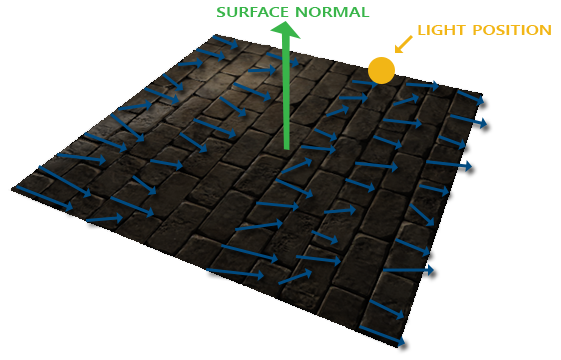## 切线空间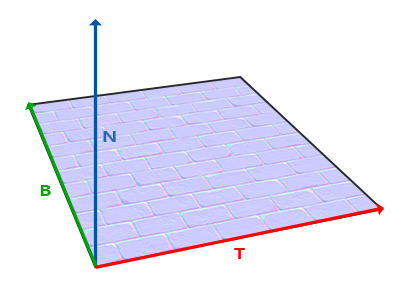$E$是两个向量位置的差，$\Delta U$$\Delta V$是纹理坐标的差。然后我们得到两个未知数（切线$T$和副切线$B$）和两个等式。你可能想起你的代数课了，这是让我们去解$T$$B$

### 手工计算切线和副切线

// positions
glm::vec3 pos1(-1.0,  1.0, 0.0);
glm::vec3 pos2(-1.0, -1.0, 0.0);
glm::vec3 pos3(1.0, -1.0, 0.0);
glm::vec3 pos4(1.0, 1.0, 0.0);
// texture coordinates
glm::vec2 uv1(0.0, 1.0);
glm::vec2 uv2(0.0, 0.0);
glm::vec2 uv3(1.0, 0.0);
glm::vec2 uv4(1.0, 1.0);
// normal vector
glm::vec3 nm(0.0, 0.0, 1.0);


glm::vec3 edge1 = pos2 - pos1;
glm::vec3 edge2 = pos3 - pos1;
glm::vec2 deltaUV1 = uv2 - uv1;
glm::vec2 deltaUV2 = uv3 - uv1;


GLfloat f = 1.0f / (deltaUV1.x * deltaUV2.y - deltaUV2.x * deltaUV1.y);

tangent1.x = f * (deltaUV2.y * edge1.x - deltaUV1.y * edge2.x);
tangent1.y = f * (deltaUV2.y * edge1.y - deltaUV1.y * edge2.y);
tangent1.z = f * (deltaUV2.y * edge1.z - deltaUV1.y * edge2.z);
tangent1 = glm::normalize(tangent1);

bitangent1.x = f * (-deltaUV2.x * edge1.x + deltaUV1.x * edge2.x);
bitangent1.y = f * (-deltaUV2.x * edge1.y + deltaUV1.x * edge2.y);
bitangent1.z = f * (-deltaUV2.x * edge1.z + deltaUV1.x * edge2.z);
bitangent1 = glm::normalize(bitangent1);

[...] // 对平面的第二个三角形采用类似步骤计算切线和副切线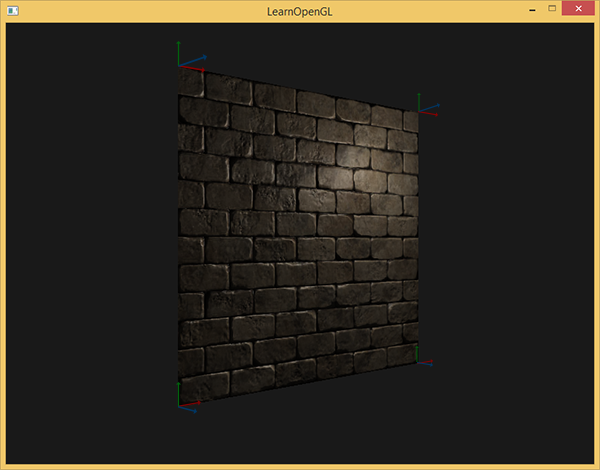### 切线空间法线贴图

#version 330 core
layout (location = 0) in vec3 position;
layout (location = 1) in vec3 normal;
layout (location = 2) in vec2 texCoords;
layout (location = 3) in vec3 tangent;
layout (location = 4) in vec3 bitangent;


void main()
{
[...]
vec3 T = normalize(vec3(model * vec4(tangent,   0.0)));
vec3 B = normalize(vec3(model * vec4(bitangent, 0.0)));
vec3 N = normalize(vec3(model * vec4(normal,    0.0)));
mat3 TBN = mat3(T, B, N)
}


Important

1. 我们直接使用TBN矩阵，这个矩阵可以把切线坐标空间的向量转换到世界坐标空间。因此我们把它传给片段着色器中，把通过采样得到的法线坐标左乘上TBN矩阵，转换到世界坐标空间中，这样所有法线和其他光照变量就在同一个坐标系中了。
2. 我们也可以使用TBN矩阵的逆矩阵，这个矩阵可以把世界坐标空间的向量转换到切线坐标空间。因此我们使用这个矩阵左乘其他光照变量，把他们转换到切线空间，这样法线和其他光照变量再一次在一个坐标系中了。

out VS_OUT {
vec3 FragPos;
vec2 TexCoords;
mat3 TBN;
} vs_out;

void main()
{
[...]
vs_out.TBN = mat3(T, B, N);
}


in VS_OUT {
vec3 FragPos;
vec2 TexCoords;
mat3 TBN;
} fs_in;


normal = texture(normalMap, fs_in.TexCoords).rgb;
normal = normalize(normal * 2.0 - 1.0);
normal = normalize(fs_in.TBN * normal);


vs_out.TBN = transpose(mat3(T, B, N));


void main()
{
vec3 normal = texture(normalMap, fs_in.TexCoords).rgb;
normal = normalize(normal * 2.0 - 1.0);

vec3 lightDir = fs_in.TBN * normalize(lightPos - fs_in.FragPos);
vec3 viewDir  = fs_in.TBN * normalize(viewPos - fs_in.FragPos);
[...]
}


out VS_OUT {
vec3 FragPos;
vec2 TexCoords;
vec3 TangentLightPos;
vec3 TangentViewPos;
vec3 TangentFragPos;
} vs_out;

uniform vec3 lightPos;
uniform vec3 viewPos;

[...]

void main()
{
[...]
mat3 TBN = transpose(mat3(T, B, N));
vs_out.TangentLightPos = TBN * lightPos;
vs_out.TangentViewPos  = TBN * viewPos;
vs_out.TangentFragPos  = TBN * vec3(model * vec4(position, 0.0));
}


glm::mat4 model;
model = glm::rotate(model, (GLfloat)glfwGetTime() * -10, glm::normalize(glm::vec3(1.0, 0.0, 1.0)));
glUniformMatrix4fv(modelLoc 1, GL_FALSE, glm::value_ptr(model));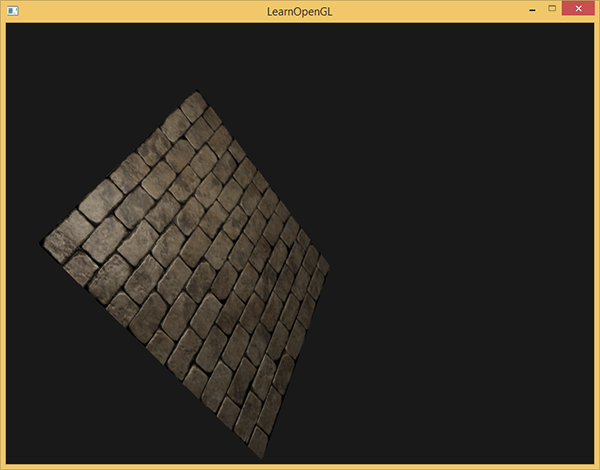### 复杂物体

const aiScene* scene = importer.ReadFile(
path, aiProcess_Triangulate | aiProcess_FlipUVs | aiProcess_CalcTangentSpace
);


vector.x = mesh->mTangents[i].x;
vector.y = mesh->mTangents[i].y;
vector.z = mesh->mTangents[i].z;
vertex.Tangent = vector;


vector<Texture> specularMaps = this->loadMaterialTextures(
material, aiTextureType_HEIGHT, "texture_normal"
);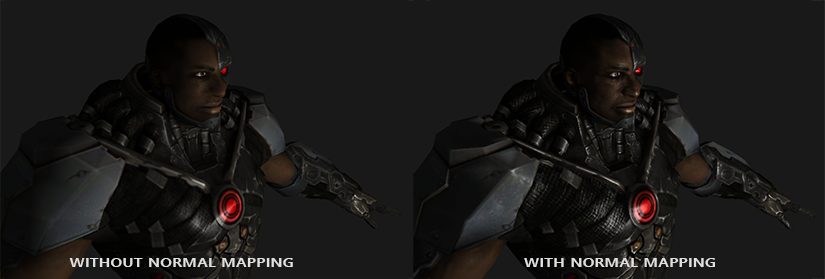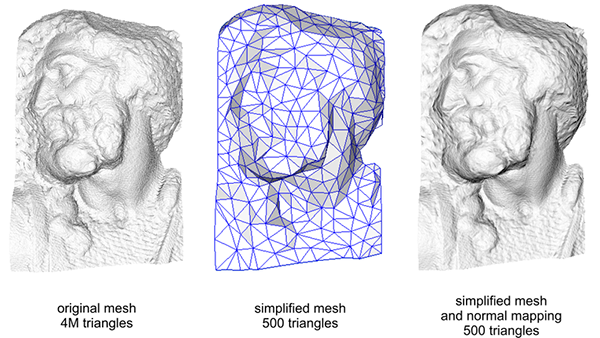## 最后一件事

vec3 T = normalize(vec3(model * vec4(tangent, 0.0)));
vec3 N = normalize(vec3(model * vec4(normal, 0.0)));
// re-orthogonalize T with respect to N
T = normalize(T - dot(T, N) * N);
// then retrieve perpendicular vector B with the cross product of T and N
vec3 B = cross(T, N);

mat3 TBN = mat3(T, B, N)